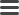# DatomiscaA Scala API for Datomic

# Building basic transaction data

## Build an assertion

Given a temporary id and a keyword corresponding to the ident of an attribute,

``````val id = DId(Partition.USER)
val attrKW = Datomic.KW(":attr")``````

we can construct an assertion as follows:

``val txData: TxData = Fact.add(id)(attrKW -> "Datomisca")``

This corresponds to:

``[:db/add id :attr "Datomisca"]``

## Build a retraction

Given an existing entity id and the `:attr` keyword from above, we can construct a retraction as follows:

``````val eId: Long = …
val txData: TxData = Fact.retract(eid)(attrKW -> "Datomisca")``````

This corresponds to:

``[:db/retract eId :attr "Datomisca"]``

## Build a partition assertion

The `Partition` class is nothing more than a value class wrapper for a `Keyword`. It purpose is simply to signal intent. We can construct a keyword intended as a partition ident and the transactiondata to assert the partition as follows:

``````val partition = new Partition(Datomic.KW(":mypartition"))
val txData: TxData = Fact.partition(partition)``````

This corresponds to:

``````{:db/id #db/id [:db.part/db]
:db/ident :mypartition
:db.install/_partition :db.part/db}``````

## Build an entity assertion

Given a temporary id and two keywords corresponding to the idents of two attributes,

``````val id = DId(Partition.USER)
val attr1KW = Datomic.KW(":attr1")
val attr2KW = Datomic.KW(":attr2")``````

we can construct an entity assertion as follows:

``````val txData: TxData =
attr1KW -> "Datomisca",
attr2KW -> "Datomic"
)``````

This corresponds to:

``````{:db/id id
:attr1 "Datomisca"
:atrr2 "Datomic"}``````

## Build an ident entity assertion

The following is a shortcut for building basic ident entities, in the case of building small enumerations:

``val txData: TxData = AddIdent(Datomic.KW(":myident"))``

This corresponds to:

``````{:db/id #db/id [:db.part/user]
:db/ident :myident}``````

Note that one can also supply a partition explicitly to `AddIdent` as a second argument.

## Build an entity retraction

Given an existing entity id, we can construct a retraction of the entire entity and all references to it as follows:

``````val eId: Long = …
Entity.retract(eId)``````

This corresponds to:

``[:db.fn/retractEntity eId]``

# Building transaction data with schema support

## Build a typed assertion

Given a temporary id and an attribute of type string and cardinality one,

``````val id = DId(Partition.USER)
val attr: Attribute[String, Cardinality.one.type] =
Attribute(
Datomic.KW(":attr"),
SchemaType.string,
Cardinality.one)``````

we can construct a typed assertion as follows:

``val txData: TxData = SchemaFact.add(id)(attr -> "Datomisca")``

A type ascription has been given to `attr` for clarity. Attributes are typed with their value type and their cardinality. This type information is used here to statically check that the type of the Scala value given is permissable. The attribute here has a value type of `String` and the value given is a string, so this expression will type check.

Ultimately, the same transaction data is generated as for:

``Fact.add(id)(attr.ident -> "Datomisca")``

## Build a typed retraction

Given an existing entity id and the attribute from above, we can construct a retraction as follows:

``````val eId: Long = …
val txData: TxData = SchemaFact.retract(eid)(attr -> "Datomisca")``````

The types are handled in exactly the same way as for assertions.

## Build a typed entity assertion

Given a temporary id, an attribute of type string and cardinality one, and an attribute of type long and cardinality one,

``````val id = DId(Partition.USER)
val attr1: Attribute[String, Cardinality.one.type] =
Attribute(
Datomic.KW(":attr1"),
SchemaType.string,
Cardinality.one)
val attr2: Attribute[Long, Cardinality.one.type] =
Attribute(
Datomic.KW(":attr2"),
SchemaType.long,
Cardinality.one)``````

we can construct a typed entity assertion as follows:

``````val txData: TxData = (
SchemaEntity.newBuilder
+= (attr1 -> "Datomisca")
+= (attr2 -> 2L)
) withId id``````

This construction is an adaptation of the collection builders from Scala’s collections library. A schema entity builder allows one to mutably build up transaction data for an entity and then seal it into an immutable result at the end.

There are some additional methods on schema entity builders that is illustrated by the following snippet:

``````val attr2: Attribute[Long, Cardinality.many.type] =
Attribute(
Datomic.KW(":attr3"),
SchemaType.long,
Cardinality.many)